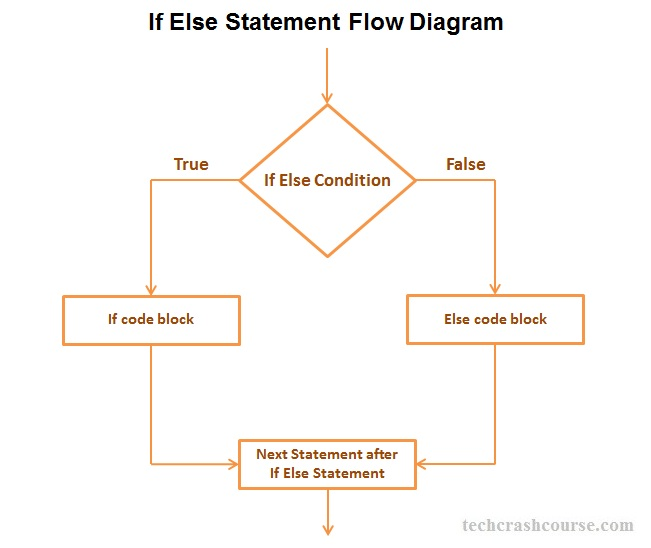# C++ If Else Statement

The if else statement in C++ programming language execute if block statements if condition is true and execute else block statements when condition is false.

Syntax of if else statement
if(condition_expression) {
// statements to be execute if the condition_expression is true
} else {
// statements to be execute if the condition_expression is false
}
• Condition written inside If statement parenthesis must be a boolean expression.
• Either if block or else block gets executed(not both) depending on the outcome of conditional expression. Both if and else block of statements can never execute at a time.
• If the condition_expression evaluated to true, then the if block of code gets executed.
• If condition_expression evaluated to false, then the block of code inside the if body is ignored and block of code inside the else body is executed.## C++ If Else Statement Example Program

```#include <iostream>
using namespace std;

int main() {
int score;
cout << "Enter your score : ";
cin >> score;
/* Using if else statement to decide whether
a student passed or failed in examination */
if(score < 35){
// if condition is true then print the following
cout << "You Failed :(\n";
} else {
// if condition is false then print the following
cout << "Congrats You Passed :)\n";
}
// This line always gets printed
cout << "Your score : " << score;
return 0;
}
```

Output
```Enter your score : 25
You Failed :(
```
```Enter your score : 60
Congrats You Passed :)
```

Points to Remember about If Else Statement
• If else statement allows the program to select one of the two action based upon the user's input or the result of an expression. It gives us flexibility to change the logic of a program in different situations.
• The condition_expression must be a boolean expression. It must evaluate to true or false value(In C++, all non-zero values are considered as true and zero is considered as false).
• We may use more than one condition inside if else statement. We can use relational and logical operators like >, <, ==, && etc to create compound boolean expressions.
For Example:
if(condition_one && condition_two) {
/* if code block */
} else {
/* else code block */
}
• Opening and Closing Braces are optional, If the block of code of if else statement contains only one statement.
For Example:
if(condition_one && condition_two)
statement1;
else
statement2
In above example only statement1 will gets executed if condition_expression is true otherwise statement2.